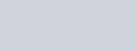Heating of 2 -chloro-1-phenylbutane with
Question:

Heating of 2 -chloro-1-phenylbutane with EtOK/EtOH gives $\mathrm{X}$ as the major product. Reaction of $\mathrm{X}$ with $\mathrm{Hg}(\mathrm{OAc})_{2} / \mathrm{H}_{2} \mathrm{O}$ followed by $\mathrm{NaBH}_{4}$ gives $\mathrm{Y}$ as the major product. $Y$ is :

1.2.3.4.Correct Option: , 4

Solution: